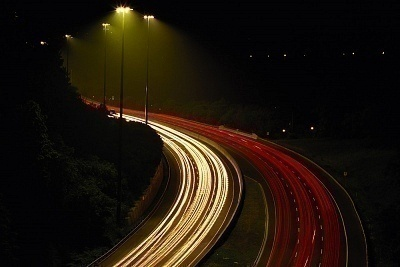•• # What is the Speed of Light in MPH?

The speed of light in MPH is 670,616,629 mph, 299,792,458 meters per second, or 186, 282 miles per second. Light takes approximately one second to travel the 238,854 miles to the moon. The speed of light is considered a physical constant that is used in many areas of physics. This is because light always travels at this speed in space or in a vacuum regardless of the measurement source or observer. The most popular use of the speed of light is in Albert Einstein’s theory of relativity, E=mc^2, where c represents the speed of light. More formally, the speed of light is the speed of a mass-less particle in a vacuum and is the upper bound on which energy or matter can travel.

## How Fast does Light Travel Through Other Surfaces?

The speed of light is less than “c” or the defined constant that travels through a vacuum when it travels through air or other materials such as glass. The ratio between the vacuum speed or “c” and that which travels through the material “v” is called the refractive index “n” of the material. “N” is equal to c/v. An example of this is that the refractive measurement of glass is classically 1.5. On the other hand, the refractive index of air is much closer to a vacuum and is equal to 1.0003 and closely approaches the value of “c.”## The History of the Speed of Light Measurement

Ole Rømer was the first person to demonstrate in 1676 that light traveled at a finite speed by studying the apparent motion of the Jupiter moon, Io. Isaac Beeckman and Galileo Galilei attempted to measure the speed of light after him, but the results were inconclusive. In 1905, Albert Einstein postulated that the speed of light in a vacuum was separate from the source of reference and demonstrated that this speed had relevance outside of the the context of electromagnetism. In 1975, the speed of light was assessed to be 299,792,458 m/s. However, the meter was redefined in 1983 as the distance light travelled in a vacuum in 1/299,792,458 second. As a result, the meter’s definition has fixed the speed of light to meters per second.

171 queries in 0.434 seconds.